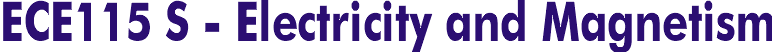Course Outline

Vector Calculus. Coulomb's and Gauss' law. Electric field of discrete and continuous charge distributions. Electric potential, energy and capacitance. Current and forces on moving charges. DC circuits. Magnetic fields. Ampère's law, Bio-Savart's formula, examples in rings and coils. Induction: Faraday's law, induced electric fields, motional emf. Example and applications: inductance. RLC transients, AC circuits and phasors.

[ECE 115S]        [ECE 424F]        [ECE1236H]       [ECE1229H]